Function Repository Resource:

# InversionCount

Count the number of pairs of out-of-order elements in a permutation

Contributed by: Wolfram Staff (original content by Sriram V. Pemmaraju and Steven S. Skiena)
 ResourceFunction["InversionCount"][p] counts the number of inversions in permutation p.

## Examples

### Basic Examples (1)

InversionCount gives the number of pairs of elements that must be reversed to bring the permutation to canonical order:

 In:=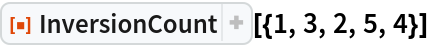Out=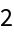In:=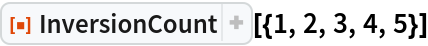Out=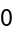In:=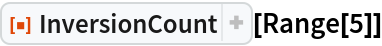Out=### Properties and Relations (7)

The number of inversions in a permutation of size n ranges from 0 to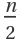:

 In:=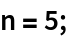In:=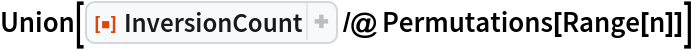Out=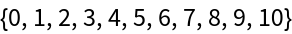In:=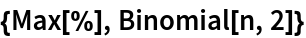Out=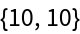The largest inversion count comes from the reverse of the identity permutation:

 In:=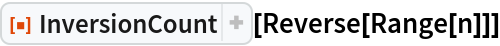Out=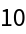There are an average of n(n-l)/4 inversions per permutation:

 In:=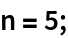In:=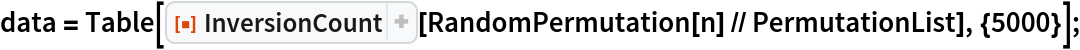In:=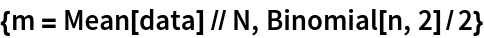Out=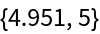In:=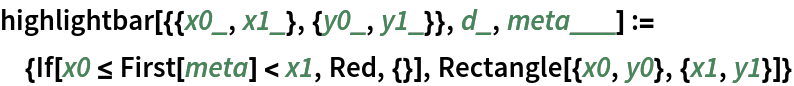In:=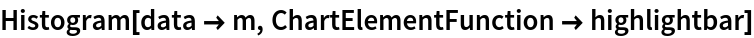Out=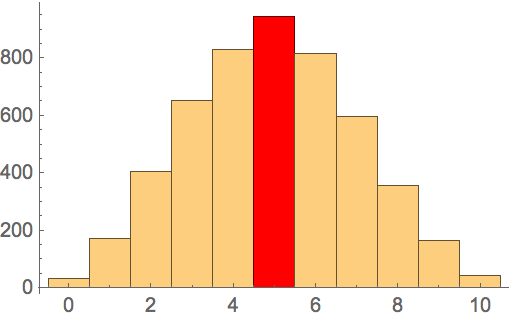The number of inversions in a permutation is equal to that of its inverse:

 In:=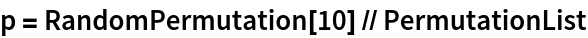Out=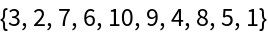In:=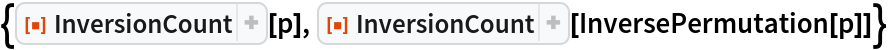Out=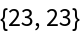The number of permutations of length n with major index k and inversion count i is the same as the number of permutations of length n with major index i and inversion count k:

 In:=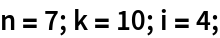In:=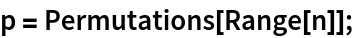In:=Out=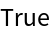The number of inversions is a sum of elements of the inversion vector:

 In:=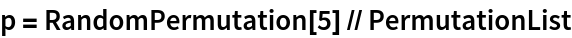Out=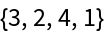In:=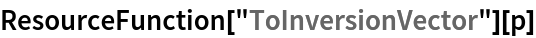Out=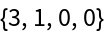In:=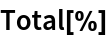Out=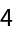In:=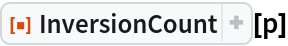Out=The number of inversions in a permutation is equal to the number of edges in its permutation graph:

 In:=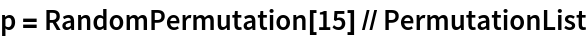Out=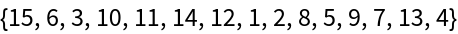In:=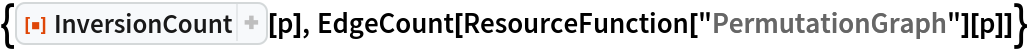Out=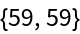The number of n permutations with k inversions is given by the resource function PermutationCountByInversions:

 In:=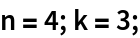In:=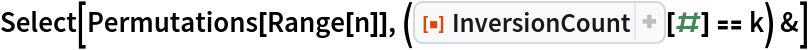Out=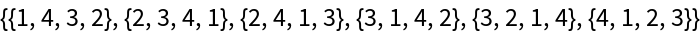In:=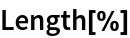Out=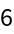In:=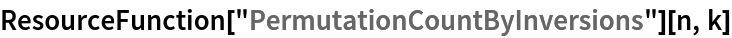Out=## Version History

• 1.0.0 – 06 July 2020